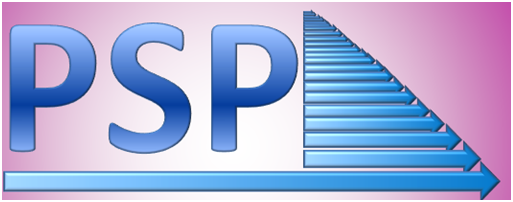Parallel Software Products        Please select sample Message Passing parallelisations of Fortran code performed by ParaWise from the menu below:

Speedup on Cray T3DSpeedup on IBM SP2# Finite Element Application Code

This Finite Element Analysis (FEA) stress/strain calculation code uses the finite element method to solve for displacement, stress and strain for two or three dimensional meshes. It allows a range of finite elements types, with differing shape functions and numbers of nodes, as well as a number of analysis modes (e.g. plain strain, axis-symmetric etc.). It uses the popular technique of constructing stiffness matrices for every finite element and incorporating contributions from each of those matrices into a global system matrix. The system matrix is then adjusted to account for boundary conditions with loadings being catered for in a right hand side of the system matrix equation. The solution of displacements to satisfy the system matrix equation is calculated using a conjugate gradient technique.

Code information: 7000 lines of source and 75 subroutines

Total Parallelization Time using ParaWise : Approximately 2 hours.

User Time: Approximately 20 minutes.

The results are for a 3000 element test case. The data partition onto 16 processors of the unstructured finite element mesh using the CAPLib library (automatically called by the parallel code generated by ParaWise) is shown below on the left. Below on the right shows a diagram of the computation solutions for stress.The same code was copied to the different parallel platforms, compiled and linked with CAPLib to create the parallel executable. The resuts to the left show good speedup and scalability of the ParaWise generated code on a Cray T3D and an IBM SP2.